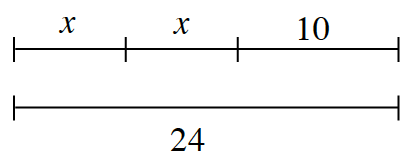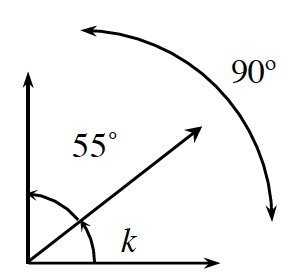### Home > CCAA > Chapter 8 Unit 7 > Lesson CC2: 8.3.1 > Problem8-61

8-61.

Write an equation that represents each diagram below and find the value of the variable.

1.How are the two lines related?

Because the two lines are equal, you can write the equation:
$x+x+10=24$

Combine like terms:
$2x+10=24$

Then solve for x:
$2x=14\\ \ \ x = 7$

$x=7$

1.Use the same method as part (a).